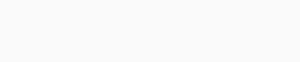# Soal Dan Kunci Jawaban Sample Paper FMO 2021 Grade 2

1. What is the next strip?A.B.C.D.E.2. Freddy uses 13 puzzle pieces to create the word FMO below. How many puzzle pieces are quadrilaterals?A. 5
B. 6
C. 7
D. 8
E. 9

3. Mr. Brown puts the number 510 into the following machine. Let’s help him calculate. What value will come out.A. 1183
B. 1093
C. 583
D. 1083
E. 1193

4. There is a pile of papers on the table. Which one is lying at the bottom?A. Red
B. Yellow
C. Orange
D. Green
E. Blue

5. Which tank is filled second?A. Tank A
B. Tank B
C. Tank C
D. Tank D
E. Tank E

6. Jimmy accidentally put some sticky tapes on his calculation and couldn’t pick it out. Let’s help Jimmy find out what the hidden digit is, given that the two hidden digits are the same.A. 7
B. 2
C. 4
D. 0
E. 8

7. Anna and Tom play the game of code. They convert all the letters into numbers
according to a rule.
If FFMOMATHRE is 1123267549 then what is FERMAT?A. 194276
B. 194267
C. 194762
D. 196427
E. 196724

8. Lucy has a sticker. She can cut her sticker into two identical pieces below.Which answer CANNOT be the shape of Lucy’s sticker?
A.B.C.D.E.9. Anna has 4 balls weighing 1kg, 2kg, 3kg, and 4kg. Anna weighed all of them and got the results as shown. How much does ball B weigh?A. 1 Kg
B. 2 Kg
C. 3 Kg
D. 4 Kg
E. 5 Kg

10. Four Girl Scouts sold cookies for one month. The graph below shows how many boxes Were sold by each Girl Scout.Which two girls sold a total of 70 boxes of cookies?
A. Isabella and Emma
B. Isabella and Grace
C. Sam and Grace
D. Sam and Emma
E. Sam and Emma

11. Lucy uses her toy blocks to build a house. Her father steps in her room and
looks at them from the right side. What does he see?A.B.C.D.E.12. .Uncle John places some bricks on top of each other to build the following flight of stairs. At least how many bricks does he have to use?A. 9
B. 11
C. 13
D. 15
E. 12

13. .A mouse is trying to escape all the traps and find the cheese. He can only
follow the lines. If the distance between two consecutive dots is 5 centimeters, what is the shortest distance he needs to travel?A. 50 cm
B. 55 cm
C. 60 cm
D. 65 cm
E. None of the above

14. John leaves home at 7 o’clock. It takes him 30 minutes to get to the restaurant. John eats breakfast for 10 minutes and then continues to go to school. After 25 minutes, he arrives at school. What time does he come to school?A.B.C.D.E.15. This pictogram shows the weather over three days in summer.Given that each symbol tells us the weather of 2 hours and 40 minutes in a day, how many hours of sunshine were there in total over the three days?
A. 6
B. 18
C. 16
D. 14
E. None of the above

16. Teacher gives each of students in class a number of candies. Anna, Tom, and Peter are talking about their candies. How many candies does Anna have?kunci jawaban: